Excel Spreadsheet Templates for Storm water Detention Pond Outlet Structure Design

Background on Storm Water Detention Ponds

Storm water retention or detention ponds are used to capture storm water runoff from a developed watershed and to release the captured storm water at a rate no greater than the pre-development peak storm water runoff rate.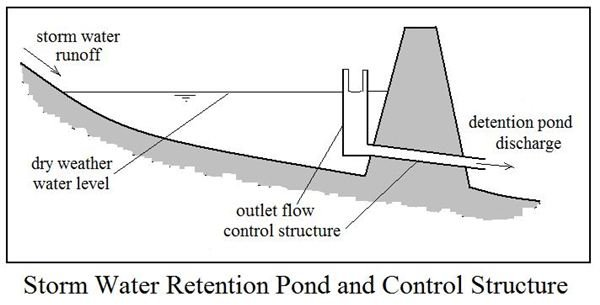The fundamental requirements are thus: i) adequate volume to hold the captured storm water runoff as it is being released at a slower rate than it is coming in, and ii) an outlet structure that controls the outflow to a rate no greater than the pre-development peak storm water runoff rate. The diagram shows a storm water detention pond and an outlet control structure with an outlet pipe and a riser with a weir.

The article, "The Storm Water Detention or Retention Pond – Why Is It Needed?" provides more background on storm water detention and retention ponds. The article "Sizing of Storm Water Detention Ponds with Excel Formulas" gives equations and Excel spreadsheets that can be downloaded to calculate the volume needed for detention/retention ponds. This article is devoted to equations and procedures for designing storm water detention/retention pond outlet structures.

Types of Outlet Control Structures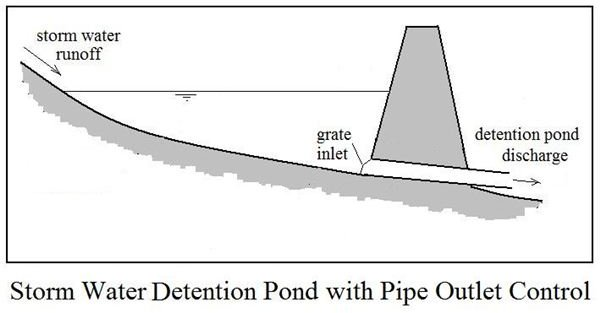In order to control the outflow from a storm water detention or retention pond to less than the pre-development peak storm water runoff rate, some type of flow constriction is needed. The three types of flow constriction/flow control devices that will be covered in this article are a pipe, an orifice, and a weir. It isn’t necessary to reinvent the wheel in order to make design calculations for a pipe, an orifice, or a weir as a flow control device, because fluid mechanics/hydraulics equations are already in common use for other applications for all three of these.A pipe of appropriate diameter and length with an inlet at or near the pond bottom can be used as the outlet control for a detention pond as shown in the diagram at the upper left. An orifice or weir outlet control will typically be used along with a riser pipe for a storm water retention pond, as shown in the diagram at the right. This type of outlet will drain the pond down only to the height of the weir crest or the bottom of the orifice after a storm event.

Single Stage and Two Stage Outlet Flow Control

The use of a pipe for the only outlet flow control, as shown in the diagram above, is an example of single stage outlet flow control. The pipe can be sized to properly restrict the flow for a storm of only one selected recurrence interval. If the pipe is sized to provide outflow at pre-development peak storm water runoff rate for a frequent event (such as a 2-yr or 5-yr storm), then it will over control less frequent events (like a 50-yr or 100-yr storm). On the other hand, if the pipe is sized to properly control a 100-yr storm, then it will under-control a more frequent event.

One solution to this dilemma is to design the outlet control for a frequent event, like the 2-yr or 5-yr storm, but provide adequate storage capacity for a less frequent event, like the 100-yr storm. Another approach is two stage outlet flow control. The weir or orifice in a riser with a pipe outlet, as shown in the diagram above can be designed for two stage control. For example, the pipe can be sized to provide the proper restriction for an event with a large recurrence interval, like a 100 year storm. The orifice or weir can then be sized to provide the proper restriction for a more frequent event, such as a 5-year storm.

The design storm recurrence interval or intervals to be used will typically be specified by a state or local agency. See page 2 for discussion and downloadable Excel spreadsheet templates to design single stage pipe outlet flow control and two stage outlet flow control with a pipe and either an orifice or a weir.

Excel Spreadsheet Templates for Pipe Outlet Control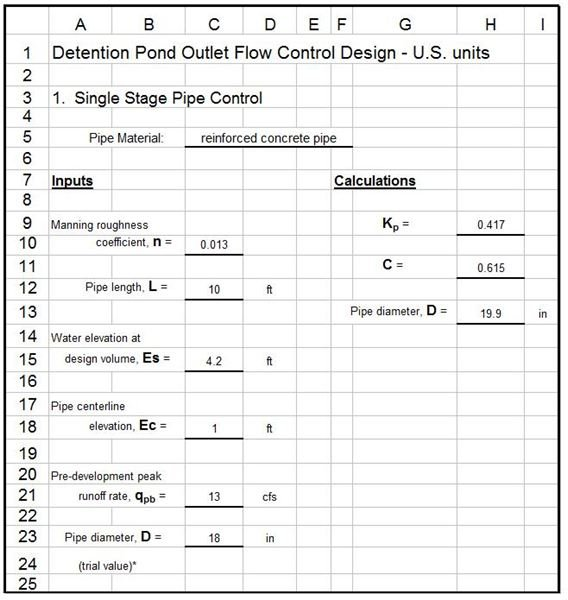The image at the left illustrates the Excel spreadsheet templates that can be downloaded to calculate the pipe diameter needed to control the outlet flow to no more than the pre-development peak storm water runoff rate for the design storm if values are provided for all of the parameters in the Inputs column. Those input parameters are shown in the diagram at the right and are summarized below:

• Manning roughness coefficient of pipe, n, dimensionless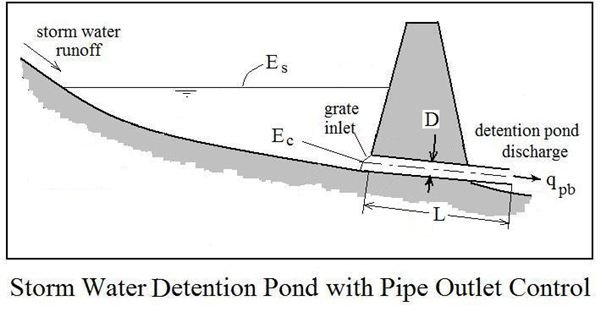• pipe length, L, ft
• water elevation in pond at design storage volume, Es, ft
• pipe centerline elevation, Ec, ft
• Pre-development peak runoff rate, qpb, cfs
• trial value for pipe diameter, D, inches

The equations used to calculate the required pipe diameter using the above parameters are:

Kp = 5087 n2 D-4/3; C = 0.456 + 0.047(L Kp) – 0.0024(L Kp)2 + 0.00006(L Kp)3; D = 12C qpb0.5(Es – Ec)-0.25

These calculations require an iterative (trial and error) solution, because a value is needed for D in order to calculate Kp, which is needed to calculate D. A trial value of D is chosen to start the process, then with values entered for all the inputs, the Excel formulas will calculate Kp, C and the pipe diameter, D. If the calculated value for D is different from the trial value, then repeat with the trial value of D set at the next standard pipe diameter less than the calculated value. Repeat if necessary.

Standard U.S. diameters for storm water drainage pipe (all in inches) are:

4, 6, 8, 12, 15, 18, 21, 24, 27, 30, 33, 36, 39, 42, 48, 54, 60, 6" increments up to 180"

Excel spreadsheet templates can be downloaded through the links below to make these calculations in either U.S. or S.I. units. The last section of this article (on page 3) gives information about the parameters and equations in S.I. units.

Excel Spreadsheet Templates for Orifice Outlet Control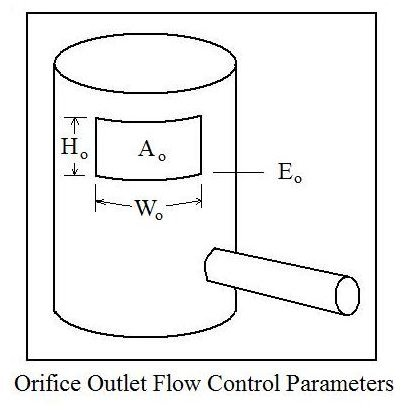The image at the left shows an orifice outlet control structure with the orifice area, Ao; width, Wo; height, Ho; and bottom elevation, Eo, shown. The image at the right below illustrates the Excel spreadsheet templates that can be downloaded to calculate the orifice size required to control the outlet flow to no more than the pre-development peak storm water runoff rate for the design storm. The Excel formulas proceed with the calculations if values are provided for the input parameters, which are only the following three:

• water elevation in pond at design storage volume, Es, ft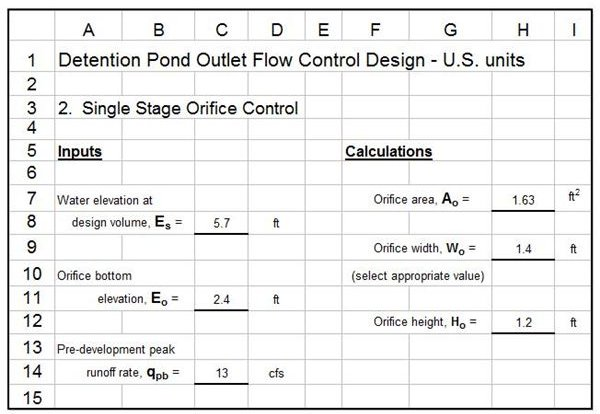• orifice bottom elevation, Ec, ft
• Pre-development peak runoff rate, qpb, cfs

The equations used by the Excel formulas to calculate the orifice height and width are as follows:

Ao = ( 0.2283 qpb )/[( Es – Eo)0.5 ] ; Ho = Ao/Wo

Two stage outlet flow control can be accomplished with an orifice as described here to control a low return period storm and a pipe designed as described in the previous section to control a high return period storm.

Excel spreadsheet templates can be downloaded through the links below to make these calculations in either U.S. or S.I. units. The last section of this article (on page 3) gives information about the parameters and equations in S.I. units.

Excel Spreadsheet Templates for Weir Outlet Control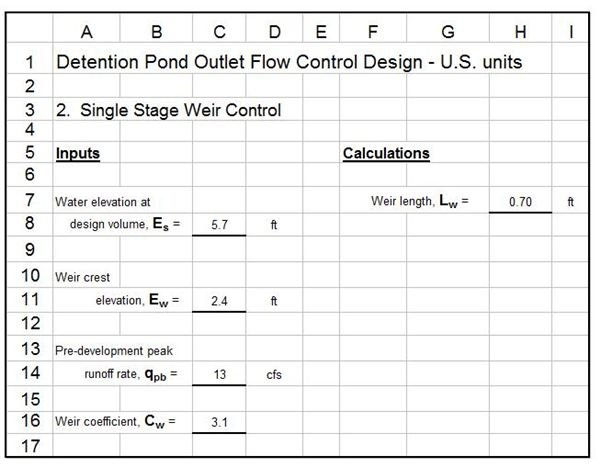The image at the left illustrates the Excel spreadsheet templates that can be downloaded to calculate the weir length needed to control the outlet flow to no more than the pre-development peak storm water runoff rate for the design storm if values are provided for the parameters in the Inputs column. Those input parameters are shown in the diagram at the right and are summarized below

• water elevation in pond at design storage volume, Es, ft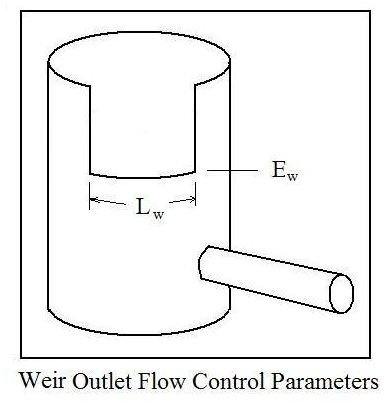• weir crest elevation, Ew, ft
• Pre-development peak runoff rate, qpb, cfs
• weir coefficient, Cw

The equation used by the Excel formulas to calculate the weir length is:

Lw = qpb/[ Cw(Es – Ew)1.5 ]

A value often used for the weir coefficient, Cw, is 3.1

Two stage outlet flow control can be accomplished with a weir as described here to control a low return period storm and a pipe designed as described in a previous section to control a high return period storm.

Excel spreadsheet templates can be downloaded through the links below to make these calculations in either U.S. or S.I. units. The next section of this article gives information about the parameters and equations in S.I. units.

S.I. units for Outlet Flow Control Design Parameters and Equations

Pipe Outlet Flow Control: The S.I. units for the parameters used with pipe outlet flow control calculations are:

• Manning roughness coefficient of pipe, n, dimensionless
• pipe length, L, m
• water elevation in pond at design storage volume, Es, m
• pipe centerline elevation, Ec, m
• Pre-development peak runoff rate, qpb, m3/s
• trial value for pipe diameter, D, mm

The equations remain nearly the same as with U.S. units, but some of the constants change, giving the following:

Kp = 115,768 n2 D-4/3 ; C = 0.456 + 0.047(LKp) – 0.0024(LKp)2 + 0.00006(LKp)3 ;

D = 1346 C qpb0.5(Es – Ec)-0.25

Orifice Outlet Flow Control: The parameters needed for orifice sizing and their S.I. units are as follows:

• water elevation in pond at design storage volume, Es, m
• orifice bottom elevation, Ec, m
• Pre-development peak runoff rate, qpb, m3/s
• Ao = orifice area, m2
• Wo = orifice width, m
• Ho = orifice height, m

The equations for S.I. units are:

Ao = 0.4135 qpb/(Es – Eo)0.5 ; Ho = Ao/Wo

Weir Outlet Flow Control: The parameters for weir outlet flow control calculations and their S.I. units are:

• water elevation in pond at design storage volume, Es, m
• Weir crest elevation, Ew, m
• Pre-development peak runoff rate, qpb, m3/s
• Weir coefficient, Cw
• Length of weir, Lw, m

The equation for calculating the required weir length remains the same ( Lw = qpb/[ Cw(Es – Ew)1.5 ], but the value typically used for the weir coefficient, Cw, is 1.71, instead of the 3.1 value used for U.S. units.

References and Image Credits

References for Further Information:

1. McCuen, Richard H., Hydrologic Analysis and Design, 2nd Ed, Upper Saddle River, NJ, 1998.

2. Coefield, S. and Environmental Health News, "EPA to Staunch Flood of Storm Water Runoff Polluting U.S. Waterways," Scientific American, February 17, 2010.

3. Mays, L. W., Urban Stormwater Management Tools, New York, McGraw-Hill, 2004.

Image Credits:

All images drawn by H. Bengtson

This post is part of the series: Storm Water Retention and Detention Ponds

Storm water retention/detention ponds are needed on a developed watershed to capture storm water runoff and release it at a rate no greater than the pre-development peak storm water runoff rate. Time of concentration and runoff coefficient are important watershed parameters